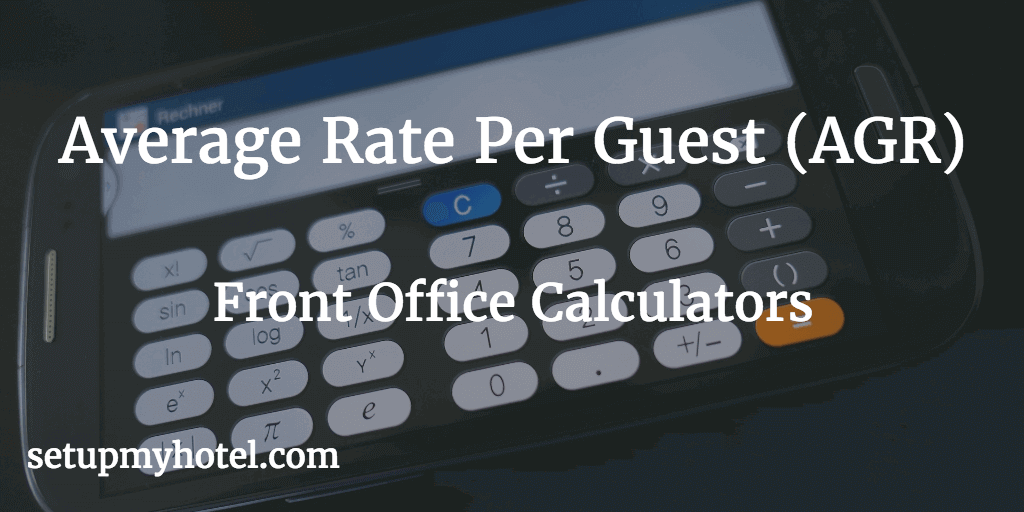# Formula For Average Rate Per Guest (AGR) - AGR Calculator

The Average Rate Per Guest (AGR) - Provides the average revenue contribution by each guest occupied in the hotel, This rate is normally based on every guest in the hotel including children. Some hotels take their AGR without considering children.

The formula for calculating Average Rate Per Guest (AGR)

Average Rate Per Guest= Total Room Revenue / Total Number of guests

Average Rate Per Guest W/O Child = Total Room Revenue / Total Number of Adults

APR - Average Guest Per Room Calculator

Total Rooms Revenue:

Total No. Of Guests:

Total No. Of Child:

APR with Total Guests =

APR Without (W/O) Child =

Example 1 AGR with Total Guests:

Total Guest Occupied for 12th September 2017 = 453

Total Room Revenue for 12th September 2017 = 70000.00

Average Rate Per Guest = 70000 / 453

= 154.53

Example 2 AGR Without (W/O) Child:

Total Adults Occupied for 12th September 2017 = 410

Total Room Revenue for 12th September 2017 = 70000.00

Average Rate Per Guest = 70000 / 410

= 170.73

Example 3 AGR with Total Guests (Monthly):

Total Guest Occupied for September 2017 = 11935

Total Room Revenue for September 2017 = 1926000.00

Average Rate Per Guest =  1926000.00 / 11935

= 161.37

Example 4 AGR Without (W/O) Child (Monthly):

Total Adults Occupied for September 2017 = 11100

Total Room Revenue for September 2017 = 1926000.00

Average Rate Per Guest = 1926000.00 / 11100

= 173.51